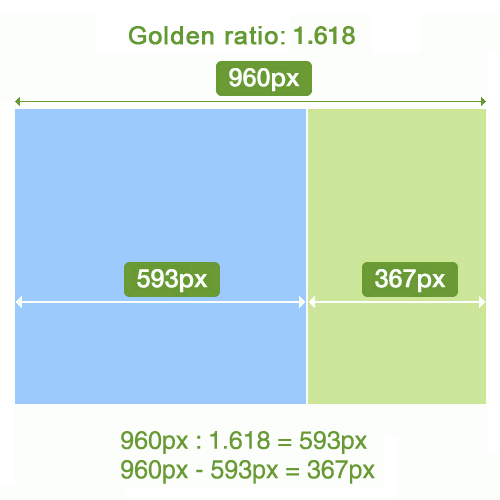# Golden ratioThe golden ratio (which is also called divine proportion, divine section, golden cut and mean of Phidias) is an irrational mathematical constant with a value of approximately Phi=1.618. If the ratio of the sum of the quantities to the larger quantity is equal to the ratio of the larger quantity to the smaller one, then the quantities are said to have a golden ratio.
You may use the ratio 5:3 which is not exactly the Golden ratio, but close to it - 1.6667.

Example:
a+b=1000
a=618
b=382

a+b=1300
a=800
b=500

a+b=800
a=500
b=300